# Precise multiplications with the NEF¶

Jan Gosmann

## Abstract¶

This report discusses how to implement the multiplication of two numbers in the NEF with a high accuracy. The main improvement will be achieved by using diagonal encoders instead of randomly distributed encoders. In the selection of the evaluation points a trade-off is found as they cannot give a uniform distribution when projected to the encoders while being limited to the input domain. That leads to an alternative multiplication network architecture improving the accuracy further.

In :
import nengo
import numpy as np
import matplotlib.pyplot as plt
from matplotlib.ticker import MaxNLocator
from scipy.stats import mannwhitneyu

%matplotlib inline


## A benchmark¶

To compare different methods of multiplication we will first define a benchmark used throughout this report. We assume that the product of two independent scalars in the range $[-1, 1]$ has to be calculated. This is a reasonable default assumption as $[-1, 1]$ is the default representational range used in the NEF and without additional information there is no reason to assume any correlation of the input values. If, however, the distribution of inputs violates this assumption (e.g. they fall into a unit circle instead of a unit square), the results in this report might not hold.

For the benchmark the whole range from $[-1, 1]$ should be covered for both values. Also, a number of combinations of different values should occur. Both values should be continuous over time because dicontinuities will introduce errors not related to the calculation of the product itself. A class of functions fulfilling these conditions is known as space-filling curves. A specific and well-known instance of such a function is the Hilbert curve. Here we define a Python class for precomputing the Hilbert curve $H_n(u), [0, 1] \rightarrow [0, 1]^2$ with a given number of iterations $n$. For $n \rightarrow \infty$ this curve will fill the complete two-dimensional input space. The start and end point of the curve are $H_n(0) = (0, 0)^{\top}$ and $H_n(1) = (1, 0)^{\top}$.

In :
class HilbertCurve(object):
# Implementation based on http://en.wikipedia.org/w/index.php?title=Hilbert_curve&oldid=633637210

def __init__(self, n):
self.n = n
self.n_corners = (2 ** n) ** 2
self.corners = np.zeros((self.n_corners, 2))
self.steps = np.arange(self.n_corners)

steps = np.arange(self.n_corners)
for s in 2 ** np.arange(n):
r = np.empty_like(self.corners, dtype='int')
r[:, 0] = 1 & (steps // 2)
r[:, 1] = 1 & (steps ^ r[:, 0])
self._rot(s, r)
self.corners += s * r
steps //= 4

self.corners /= (2 ** n) - 1

def _rot(self, s, r):
swap = r[:, 1] == 0
flip = np.all(r == np.array([1, 0]), axis=1)

self.corners[flip] = (s - 1 - self.corners[flip])
self.corners[swap] = self.corners[swap, ::-1]

def __call__(self, u):
step = np.asarray(u * len(self.steps))
return np.vstack((
np.interp(step, self.steps, self.corners[:, 0]),
np.interp(step, self.steps, self.corners[:, 1]))).T


The next code cell defines some benchmarking parameters. The duration should not be too low. Otherwise the main contribution to the measured error will be the time lag of the neural implementation instead of the error in the representation. However, increasing the duration will also increase simulation times.

In :
master_seed = 1298      # Seed for generation of individual seeds for trials
n_trials = 50           # Number of trials
hc = HilbertCurve(n=4)  # Increase n to cover the input space more densly
dt = 0.001              # Simulation time step (seconds)
duration = 5.           # Simulation duration (seconds)
wait_duration = 0.5     # Duration (seconds) to wait in the beginning to have stable representation of the initial values.

def gen_seeds(size):
return np.random.RandomState(master_seed).randint(nengo.utils.numpy.maxint, size=size)

seeds = gen_seeds(n_trials)   # Random number generator seeds for reproducibility


The Hilbert curve will be in the range of $[0, 1]^2$, but the actual input will be in $[-1, 1]^2$. Thus, some scaling has to be applied. Also, $u$ has to be scaled to the duration of a trial.

In :
def stimulus_fn(t):
return np.squeeze(hc(t / duration).T * 2 - 1)


This is what the input and desired output look like:

In :
ts = np.linspace(0, duration, duration / dt)
inp = stimulus_fn(ts)

plt.figure(figsize=(8, 3))

plt.subplot(1, 2, 1, aspect='equal')
plt.plot(*inp)
plt.title("Bechmark input")
plt.xlabel("$x_1$")
plt.ylabel("$x_2$")
plt.xlim(-1.1, 1.1)
plt.ylim(-1.1, 1.1)

plt.subplot(1, 2, 2)
plt.plot(ts, np.prod(inp, axis=0))
plt.title("Desired output")
plt.xlabel("t [s]")
plt.ylabel("y");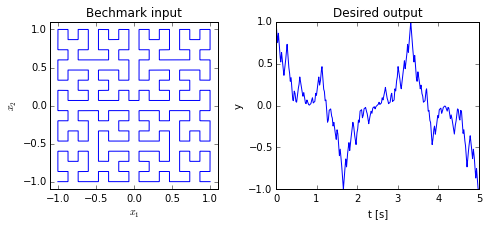We use this input to feed it into the product network under test. From the decoded output and an ensemble calculating the same product in direct mode the root mean square error (RMSER) is computed as a performance indicator.

In :
from nengo.utils.numpy import rmse

class Benchmark(object):
def __init__(self, seed, create_mult_network, test_config, synapse=None, n_neurons=150, n_eval_points=1000):
self.n_neurons = n_neurons
self.n_eval_points = n_eval_points
self.seed = seed

model = nengo.Network(seed=seed)
with model:
model.config[nengo.Probe].synapse = synapse
model.config[nengo.Connection].synapse = synapse

stimulus = nengo.Node(output=lambda t: stimulus_fn(max(0., t - wait_duration)), size_out=2)

with test_config:
self.probe_test = create_mult_network(self.n_neurons, self.n_eval_points, stimulus)

ens_direct = nengo.Ensemble(1, dimensions=2, neuron_type=nengo.Direct())
result_direct = nengo.Node(size_in=1)
nengo.Connection(stimulus, ens_direct)
nengo.Connection(ens_direct, result_direct, function=lambda x: x * x, synapse=None)
self.probe_direct = nengo.Probe(result_direct)

self.sim = nengo.Simulator(model)
self.sim.run(duration + wait_duration, progress_bar=False)

self.trange = self.sim.trange()[self.sim.trange() > wait_duration] - wait_duration
self.output_test = self.sim.data[self.probe_test][self.sim.trange() > wait_duration]
self.output_direct = self.sim.data[self.probe_direct][self.sim.trange() > wait_duration]

def rmse(self):
return rmse(self.output_test, self.output_direct)

def plot(self):
ax = plt.gca()
ax.plot(self.trange, self.output_test, label="Test")
ax.plot(self.trange, self.output_direct, label="Direct")
ax.legend(loc='best')
ax.set_xlabel("t [s]")
ax.set_ylabel("y")
ax.set_xlim(0., 1.)
ax.set_ylim(-1.1, 1.1)

In :
def repeated_benchmark(*args, **kwargs):
rmses = np.empty(len(seeds))
with nengo.utils.progress.ProgressTracker(len(seeds), True) as progress:
for i, s in enumerate(seeds):
b = Benchmark(s, *args, **kwargs)
rmses[i] = b.rmse()
if i == 0:
b.plot()
progress.step()
print "mean RMSE:", np.mean(rmses)
print "median RMSE:", np.median(rmses)
print "standard deviation of RMSE:", np.std(rmses)
return rmses


### The Naive implementation¶

To start out we will use a single ensemble and we will directly decode the multiplication result from it. Note that a radius of $\sqrt{2}$ is used to ensure all possible input values fall into the representational range of the ensemble.

In :
def naive_multiplication(n_neurons, n_eval_points, stimulus):
# Note that we set the radius to sqrt(2.) to make sure all possible input values
# are within the representational range of the ensemble. (Both multiplicands
# could be 1, giving a distance of sqrt(1²+1²) to the origin.)
ens = nengo.Ensemble(n_neurons, dimensions=2, radius=np.sqrt(2.), n_eval_points=n_eval_points)
result = nengo.Node(size_in=1)
nengo.Connection(stimulus, ens)
nengo.Connection(ens, result, function=lambda x: x * x, synapse=None)
return nengo.Probe(result)


To obtain first benchmark results LIF rate neurons will be used. This allows us to solely look at the representational error without considering the spiking noise. Because this means overall less noise in the network, the decoder solver regularization is reduced to 0.01. Also, all synapses are set to None to remove any filtering and time lag.

With this configuration we obtain a baseline error with the naive implementation.

In :
config = nengo.Config(nengo.Connection, nengo.Ensemble)
config[nengo.Ensemble].neuron_type = nengo.LIFRate()
config[nengo.Connection].solver = nengo.solvers.LstsqL2(reg=0.01)

rmse_naive = repeated_benchmark(naive_multiplication, config)

mean RMSE: 0.0131866845469
median RMSE: 0.0132555941685
standard deviation of RMSE: 0.00147545718196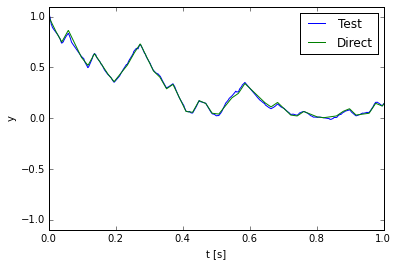We use the following function to compare different results:

In :
def compare(before, after):
print "Improvement by {0:.0f}% (p < {1:.3f}).".format(
(1. - np.mean(after) / np.mean(before)) * 100.,
np.ceil(1000. * 2. * mannwhitneyu(before, after)) / 1000.)


## Encoders¶

The largest reduction in error is obtained by using diagonal encoders. To see why this is the case we analytically derive the error in different directions of the input space.

Assume that the multiplicands are given as a vector $\vec x = (x_1, x_2)^{\top}$. Then the result of the product can be rewritten in polar coordinates:

$$x_1 x_2 = r^2 \cos \varphi \sin \varphi$$

Furthermore, we assume a special neuron model in the derivations to simplify the math For every input on its encoder $\vec e = (e_1, e_2)^{\top}, |\vec e| = 1$ its activity is a perfect product, but the input current proportional to $\vec e \cdot \vec x$ is rectified:

$$a(\vec x) = G(\vec e \cdot \vec x) = e_1 [\vec e \cdot \vec x]_+ e_2 [\vec e \cdot \vec x]_+$$

Such a neuron can be approximated by with an ensemble LIF neurons and thus the following derivation also holds for LIF neurons.

With $\psi$ as the polar coordinate of the encoder $\vec e$ this can be rewritten as

$$a(\vec x) = r^2 \cos \psi \sin \psi [\cos(\psi - \varphi)]_+^2$$

This allows to write down the error for multiplications with multiplicands aligned along $\varphi$. As we are using polar coordinates an additional $r$ appears in the integral.

$$E^2(\varphi) = \int_0^{r(\phi)} (x_1 x_2 - \sum_i a_i(\vec x))^2\ r\ \mathrm{d}r \\ = \int_0^{r(\phi)} (r^2 \cos \varphi \sin \varphi - r^2 \sum_i \cos \psi_i \sin \psi_i [\cos(\psi_i - \varphi)]_+^2)^2\ r\ \mathrm{d}r \\ = \frac{r^6(\varphi)}{6} (\cos \varphi \sin \varphi - \sum_i \cos \psi_i \sin \psi_i [\cos(\psi_i - \varphi)]_+^2)^2$$

In this equation $r(\varphi)$ is the “radius” of the multiplication input space for a given $\varphi$. For the square input space of $[-1, 1]^2$ used here, $r(\varphi)$ is given by

$$r(\varphi) = \frac{1}{\cos([(\varphi + \frac{\pi}{4})\mod \frac{\pi}{2}] - \frac{\pi}{4})} \mathrm{.}$$
In :
def radius(phi):
return 1. / np.cos((phi + np.pi / 4.) % (np.pi / 2.) - np.pi / 4.)

phis = np.linspace(0., 2. * np.pi, 100)
ax = plt.subplot(1, 1, 1, polar=True)

Out:
[<matplotlib.lines.Line2D at 0x7fe44512bd90>]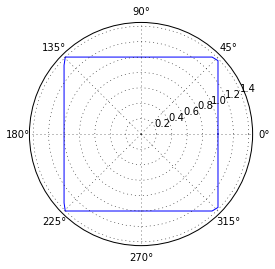In :
def mult_err(phi, encoders=[]):
if len(encoders) > 0:
cos_sin_psi = np.cos(encoders) * np.sin(encoders)
diff = np.maximum(0., np.cos(np.subtract.outer(encoders, phi)))
neuron_outputs = cos_sin_psi[:, np.newaxis] * diff ** 2.
else:
neuron_outputs = np.zeros((0, np.asarray(phi).size))
return radius(phi) ** 6. / 6. * (np.cos(phi) * np.sin(phi) - np.sum(neuron_outputs, axis=0))  ** 2.


Without any neurons we get the following distribution of the error:

In :
phis = np.linspace(0., 2. * np.pi, 100)
ax = plt.subplot(1, 1, 1, polar=True)
ax.plot(phis, mult_err(phis))
ax.set_rmax(0.35)
ax.yaxis.set_major_locator(MaxNLocator(3))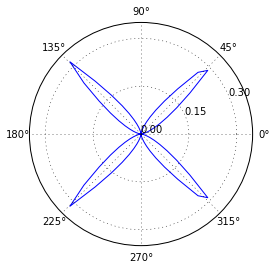As we see the maxima of the error are aligned with the diagonals. By adding neurons with each diagonal as an encoder one after another the error gets reduced to zero:

In :
encoders = [np.pi / 4., 3. / 4. * np.pi, 5. / 4. * np.pi, 7. / 4. * np.pi]

plt.figure(figsize=(20, 8))
for i in range(1, len(encoders) + 1):
ax = plt.subplot(1, len(encoders) + 1, i + 1, polar=True)
ax.plot(phis, mult_err(phis, encoders=encoders[:i]))
ax.set_rmax(0.35)
ax.yaxis.set_major_locator(MaxNLocator(3))
ax.set_title("{0} encoders".format(i))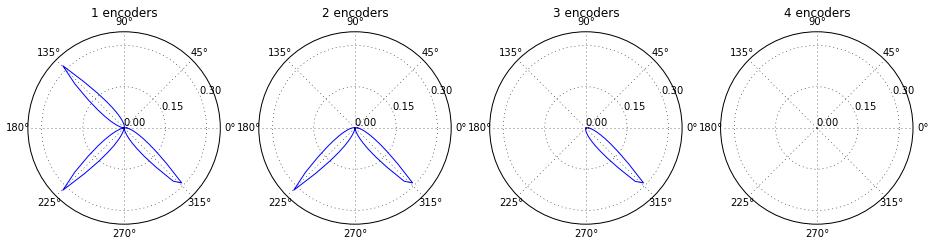Using encoders with a different alignment will give worse results as the maximal possible reduction in error is less than for the diagonal directions. With those diagonal encoders instead fo random encoders in the benchmark we obtain a substantial reduction of the error.

In :
diag_encoders = nengo.dists.Choice(np.array([[1, 1], [1, -1], [-1, -1], [-1, 1]]) / np.sqrt(2.))

config = nengo.Config(nengo.Connection, nengo.Ensemble)
config[nengo.Ensemble].neuron_type = nengo.LIFRate()
config[nengo.Connection].solver = nengo.solvers.LstsqL2(reg=0.01)
config[nengo.Ensemble].encoders = diag_encoders

rmse_diag_encoders = repeated_benchmark(naive_multiplication, config)

mean RMSE: 0.0053812936708
median RMSE: 0.00535873543816
standard deviation of RMSE: 0.000906316208863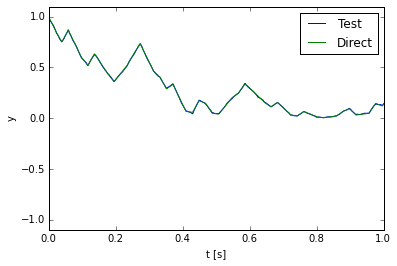In :
compare(rmse_naive, rmse_diag_encoders)

Improvement by 59% (p < 0.001).


## Evaluation points¶

The evaluation points are sampled from a circle with radius $r$ per default. As we assumed independent inputs we set $r = \sqrt{2}$ to have values up to $\vec x = (1, 1)^{\top}$ in range. This, however, will produce a number of evaluation points outside the allowed input range. Here is a visualization with the range of possible inputs as the red square and the area from which the evaluation points are chosen as blue cirle.

In :
plt.subplot(1, 1, 1, aspect='equal')
plt.gca().add_artist(plt.Rectangle([-1, -1], 2, 2, fill=False, color='r'))
plt.xlabel("$x_1$")
plt.ylabel("$x_2$")
plt.xlim(-1.5, 1.5)
plt.ylim(-1.5, 1.5);
plt.title("Input domain and sampling shape")

Out:
<matplotlib.text.Text at 0x7fe44526e210>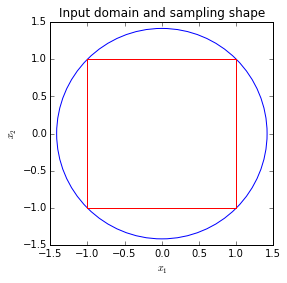In theory we can achieve a more accurate product by sampling the evaluation points from the square of actual possible inputs instead of “wasting” some in an irrelevant area.

Note that the Nengo scales the evaluation points with the ensemble radius. Because of that, it is necessary to divide the range by the radius.

In :
config = nengo.Config(nengo.Connection, nengo.Ensemble)
config[nengo.Ensemble].neuron_type = nengo.LIFRate()
config[nengo.Connection].solver = nengo.solvers.LstsqL2(reg=0.01)
config[nengo.Ensemble].eval_points = nengo.dists.Uniform(-1 / np.sqrt(2.), 1 / np.sqrt(2.))

rmse_square = repeated_benchmark(naive_multiplication, config)

mean RMSE: 0.0137222112603
median RMSE: 0.013483329981
standard deviation of RMSE: 0.00168910500221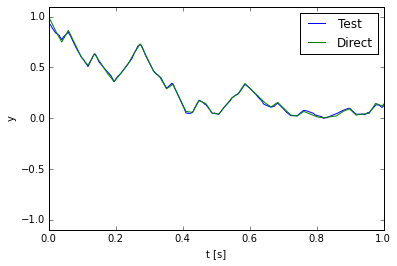In :
compare(rmse_naive, rmse_square)

Improvement by -4% (p < 0.211).


However, this change of evaluation points has no significant effect.

Surprisingly, picking evaluation points from the square performs worse if it is done together with the diagonal encoders.

In :
config = nengo.Config(nengo.Connection, nengo.Ensemble)
config[nengo.Ensemble].neuron_type = nengo.LIFRate()
config[nengo.Connection].solver = nengo.solvers.LstsqL2(reg=0.01)
config[nengo.Ensemble].encoders = diag_encoders
config[nengo.Ensemble].eval_points = nengo.dists.Uniform(-1 / np.sqrt(2.), 1 / np.sqrt(2.))

rmse_square_diag_encoders = repeated_benchmark(naive_multiplication, config)

mean RMSE: 0.00734397454231
median RMSE: 0.00701522040061
standard deviation of RMSE: 0.00173103578512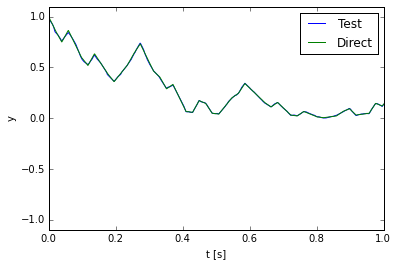In :
compare(rmse_diag_encoders, rmse_square_diag_encoders)

Improvement by -36% (p < 0.001).


### Evaluation points get projected¶

To understand this one has to realize that the evaluation points get projected onto the encoders. When we look at the distribution of projected evaluation points we see a difference comparing the square and circle as sampling shape. Evaluation points from the circle give a flatter distribution and more points project to the positive and negative end of the encoding vector.

In :
n_samples = 10000
seed = 23987
ep1 = nengo.dists.UniformHypersphere().sample(n_samples, 2, rng=np.random.RandomState(seed)) * np.sqrt(2.)
ep2 = nengo.dists.Uniform(-1, 1).sample(n_samples, 2, rng=np.random.RandomState(seed))
encoder = np.array([1, 1]) / np.sqrt(2.)

n_bins = 50
plt.hist(np.dot(ep1, encoder), alpha=0.5, bins=n_bins, label='circle')
plt.hist(np.dot(ep2, encoder), alpha=0.5, bins=n_bins, label='square')
plt.legend(loc='best');
plt.title("Projected evaluation points");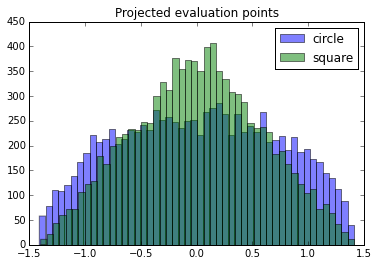Both sampling shapes, but more so the square, give more weight to values projecting to smaller magnitudes. Thus, the approximation of the function will be better in the area where it is flattest and gives the least contribution to the overall error. This leads to the hypothesis that a a distribution of evaluation points that projects to a uniform distribution could be best. A diamond shaped sampling region would be achieve this uniform distribution in the projection.

In :
class DiamondDist(nengo.dists.Distribution):
def sample(self, n, d, rng=np.random):
uniform = nengo.dists.Uniform(-2., 2.)
samples = np.empty((n, d))
for i in range(n):
samples[i] = uniform.sample(1, d)
while np.sum(np.abs(samples[i])) > 2.:
samples[i] = uniform.sample(1, d)
return samples

In :
samples = DiamondDist().sample(500, 2, rng=np.random.RandomState(seed))

plt.subplot(1, 1, 1, aspect='equal')
plt.gca().add_artist(plt.Rectangle([-1, -1], 2, 2, fill=False, color='r'))
plt.xlabel("$x_1$")
plt.ylabel("$x_2$")
plt.title("Points sampled from a diamond")
plt.xlim(-2., 2.)
plt.ylim(-2., 2.);
plt.scatter(samples[:, 0], samples[:, 1], marker='+')

Out:
<matplotlib.collections.PathCollection at 0x7fe444bf4990>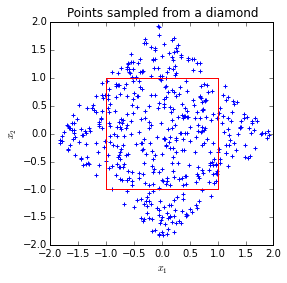In :
n_samples = 10000
ep = DiamondDist().sample(n_samples, 2, rng=np.random.RandomState(seed))
encoder = np.array([1, 1]) / np.sqrt(2.)

n_bins = 50
plt.hist(np.dot(ep, encoder), alpha=0.5, bins=n_bins)
plt.title("Projected evaluation points");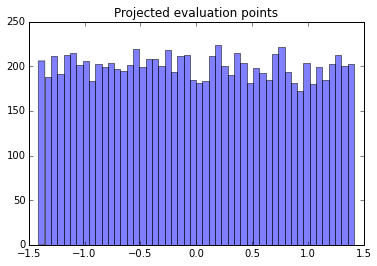In :
config = nengo.Config(nengo.Connection, nengo.Ensemble)
config[nengo.Ensemble].neuron_type = nengo.LIFRate()
config[nengo.Connection].solver = nengo.solvers.LstsqL2(reg=0.01)
config[nengo.Ensemble].encoders = diag_encoders
config[nengo.Ensemble].eval_points = DiamondDist()

rmse_diamond_diag_encoders = repeated_benchmark(naive_multiplication, config)

mean RMSE: 0.019287935708
median RMSE: 0.0192496543946
standard deviation of RMSE: 0.00229384534606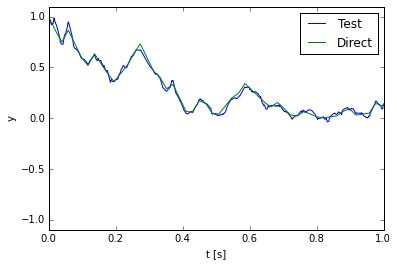In :
compare(rmse_diag_encoders, rmse_diamond_diag_encoders)

Improvement by -258% (p < 0.001).


The diamond shape is not any better. Even though it gives a flat distribution in the projection, a lot of evaluation points fall outside of the input domain. As long as we use diagonal encoders there will be a trade off between a flat distribution in the projection and having all evaluation points in the input domain. This is because we have two orthogonal encoder directions. An evaluation point within the input domain at the end of one encoder will be project close to 0 on the other encoder.

## An alternative network¶

As it is not possible to eliminate that trade off in a single ensemble we have to split it up into two. Each ensemble will be one dimensional and will represent one encoder direction. Given the input $\vec x$ and encoders $\vec e_1, \vec e_2$ on the diagonal the multiplication can be rewritten in the following way:

$$x_1 x_2 = \frac{1}{4}(x_1^2 + 2 x_1 x_2 + x_2^2) - \frac{1}{4}(x_1^2 - 2 x_1 x_2 + x_2^2) \\ = \frac{1}{4}(x_1 + x_2)^2 - \frac{1}{4}(x_1 - x_2)^2 \\ = \frac{1}{4}((1, 1)^{\top} \cdot \vec x)^2 - \frac{1}{4}((1, -1)^{\top} \cdot \vec x)^2 \\ = \frac{1}{2}[(\vec e_1 \cdot \vec x)^2 - (\vec e_2 \cdot \vec x)^2]$$

Here is the corresponding Nengo implementation:

In :
def two_ens_multiplication(n_neurons, n_eval_points, stimulus):
ens1 = nengo.Ensemble(n_neurons // 2, dimensions=1, radius=np.sqrt(2.), n_eval_points=n_eval_points)
ens2 = nengo.Ensemble(n_neurons // 2, dimensions=1, radius=np.sqrt(2.), n_eval_points=n_eval_points)
nengo.Connection(stimulus, ens1, transform=np.array([[1, 1]]) / np.sqrt(2.))
nengo.Connection(stimulus, ens2, transform=np.array([[1, -1]]) / np.sqrt(2.))
result = nengo.Node(size_in=1)
nengo.Connection(ens1, result, transform=0.5, function=np.square, synapse=None)
nengo.Connection(ens2, result, transform=-0.5, function=np.square, synapse=None)
return nengo.Probe(result)


The number of evaluation points $Q$ is not split into half to keep the number of elements in the matrices when solving for decoders the same. Those matrices are a $N \times Q$ activity matrix and a $N \times d$ target matrix wherein $N$ is the number of neurons and $d$ the number of dimensions. In the naive network this means we have one $N \times Q$ and one $N \times 2$ matrix. In the alternative network it is two matrices with $\frac{N}{2} \times Q$ elements and two matrices with $N \times 1$ element.

In :
config = nengo.Config(nengo.Connection, nengo.Ensemble)
config[nengo.Ensemble].neuron_type = nengo.LIFRate()
config[nengo.Connection].solver = nengo.solvers.LstsqL2(reg=0.01)

rmse_two_ens = repeated_benchmark(two_ens_multiplication, config)

mean RMSE: 0.00525503429933
median RMSE: 0.00504524950694
standard deviation of RMSE: 0.000769015149906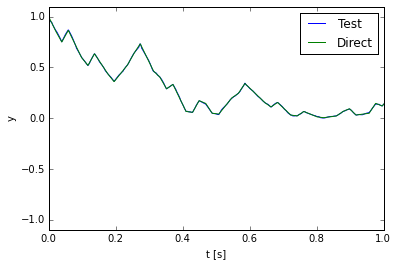In :
compare(rmse_diag_encoders, rmse_two_ens)

Improvement by 2% (p < 0.344).


The p-value is too high to reject the null hypothesis (no change in error). However, it is easy to increase the number of trials to see if we can get a clearer result.

In :
seeds = gen_seeds(300)

config = nengo.Config(nengo.Connection, nengo.Ensemble)
config[nengo.Ensemble].neuron_type = nengo.LIFRate()
config[nengo.Connection].solver = nengo.solvers.LstsqL2(reg=0.01)
config[nengo.Ensemble].encoders = diag_encoders

rmse_diag_encoders2 = repeated_benchmark(naive_multiplication, config)

config = nengo.Config(nengo.Connection, nengo.Ensemble)
config[nengo.Ensemble].neuron_type = nengo.LIFRate()
config[nengo.Connection].solver = nengo.solvers.LstsqL2(reg=0.01)

rmse_two_ens2 = repeated_benchmark(two_ens_multiplication, config)

seeds = gen_seeds(n_trials)  # Reset number of trials

mean RMSE: 0.00540763793025
median RMSE: 0.00534008366937
standard deviation of RMSE: 0.000848175504177
mean RMSE: 0.00514186445731
median RMSE: 0.00507345171245
standard deviation of RMSE: 0.000648266251299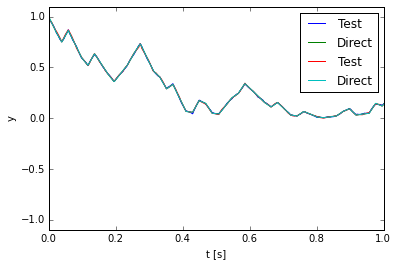In :
compare(rmse_diag_encoders2, rmse_two_ens2)

Improvement by 5% (p < 0.001).


With the large sample size we can indeed find an improvement, though only a small one.

## Using spiking neurons¶

So far the improvements have only been shown for rate neurons without any filtering. Here we will use spiking neurons and a commonly used exponential decaying synapse with a time constant of 5ms.

In :
synapse = nengo.Lowpass(0.005)

In :
config = nengo.Config(nengo.Connection, nengo.Ensemble)
rmse_naive_spiking = repeated_benchmark(naive_multiplication, config, synapse=synapse)

mean RMSE: 0.0700950596452
median RMSE: 0.069612157843
standard deviation of RMSE: 0.00749646471521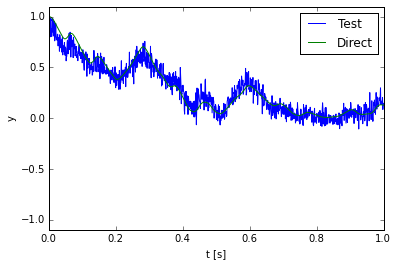In :
config = nengo.Config(nengo.Connection, nengo.Ensemble)
config[nengo.Ensemble].encoders = diag_encoders
rmse_diag_encoders_spiking = repeated_benchmark(naive_multiplication, config, synapse=synapse)

mean RMSE: 0.0481133982306
median RMSE: 0.0477134127208
standard deviation of RMSE: 0.0047371435676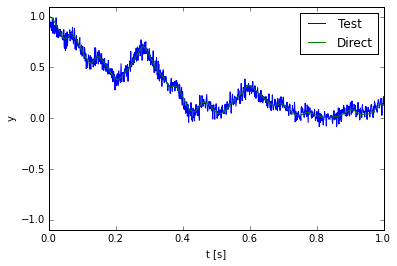In :
config = nengo.Config(nengo.Connection, nengo.Ensemble)
rmse_two_ens_spiking = repeated_benchmark(two_ens_multiplication, config, synapse=synapse)

mean RMSE: 0.0444385504631
median RMSE: 0.0445897354704
standard deviation of RMSE: 0.00399430632672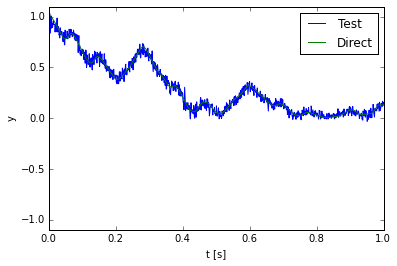In :
print "naive vs. diagonal encoders:"
compare(rmse_naive_spiking, rmse_diag_encoders_spiking)
print "diagonal encoders vs. alternative network:"
compare(rmse_diag_encoders_spiking, rmse_two_ens_spiking)
print "naive vs. alternative network:"
compare(rmse_naive_spiking, rmse_two_ens_spiking)

naive vs. diagonal encoders:
Improvement by 31% (p < 0.001).
diagonal encoders vs. alternative network:
Improvement by 8% (p < 0.001).
naive vs. alternative network:
Improvement by 37% (p < 0.001).


With the spiking neurons the improvement of the diagonal encoders is less pronounced, but still large. The alternative network performs clearly better for spiking neurons.

## Conclusion¶

The accuracy of a multiplication implemented in the NEF could be improved by 37% overall compared to the naive implementation. By considering the distribution of the error it was first derived that diagonal encoders are optimal. This insight could be used to construct an alternative more accurate multiplication network.

## Acknowlegdements¶

I thank Sam Fok for helpful comments on early versions of this report and Terry Stewart for statistical advice.

## Appendix¶

This IPython notebook is based on Nengo 2.0.0. Further version information:

In :
import matplotlib
import scipy
import sys

print "Python", sys.version
print "Nengo", nengo.version.version
print "NumPy", np.version.version
print "SciPy", scipy.version.version
print "Matplotlib", matplotlib.__version__

Python 2.7.9 (default, Jan 19 2015, 15:29:26)
[GCC 4.9.1 20140903 (prerelease)]
Nengo 2.0.0
NumPy 1.9.1
SciPy 0.15.1
Matplotlib 1.4.2

In :